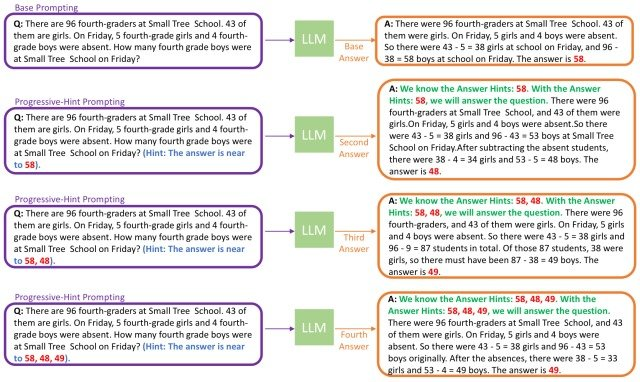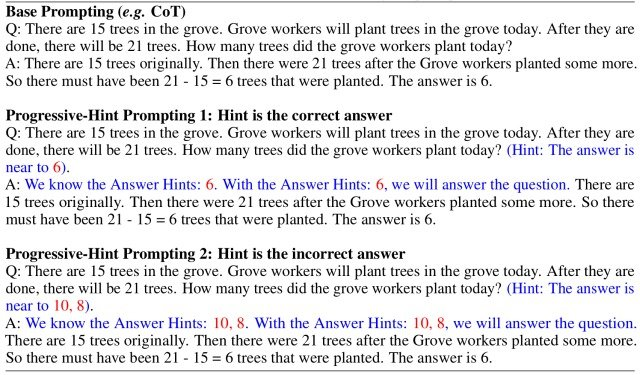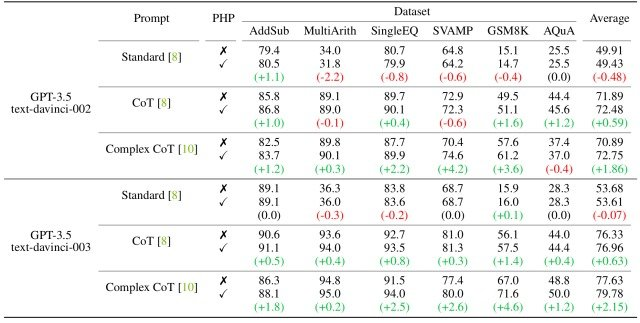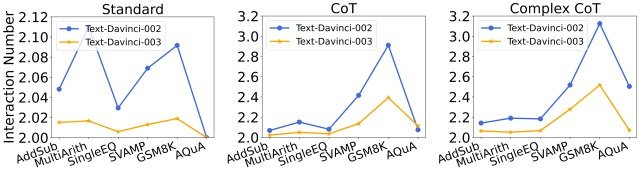# Catch up on the latest AI articles# An Approach To Improving Reasoning Ability By Giving Hints To LLMs Is Now Available!

3 main points
✔️ Propose Progressive-Hint Prompting (PHP), a prompting method that follows human thought processes and leverages past responses as hints
✔️ Demonstrate its effectiveness through comparative experiments using various data sets and prompts
✔️ The stronger the model and prompts found that PHP's performance improves the more powerful the

written by Chuanyang ZhengZhengying LiuEnze XieZhenguo LiYu Li
(Submitted on 19 Apr 2023 (v1), last revised 10 Aug 2023 (this version, v5))

Subjects: Computation and Language (cs.CL); Machine Learning (cs.LG)code：The images used in this article are from the paper, the introductory slides, or were created based on them.

## Introduction

While large-scale language models (LLMs) have demonstrated remarkable performance in a variety of natural language processing tasks, their inferential power is highly dependent on the design of the prompts.

Chain-of-Thought (CoT) and self-consistency, which have emerged in recent research, have attracted attention as important methods for enhancing these reasoning abilities, but these methods have the problem of not fully utilizing the answers generated by the LLM.

On the other hand, existing research has not examined the effectiveness of methods that iteratively improve reasoning ability by leveraging LLM outputs in the same way as human thought processes.

In this paper, we propose Progressive-Hint Prompting (PHP), a new prompting method that follows the human thought process, utilizing past answers as hints and deriving the correct answer after re-evaluating the problem. The paper demonst rates its effectiveness through comprehensive experiments using various LLMs.

## Progressive-Hint Prompting(PHP)

One of the hallmarks of the human thought process is the ability to not only think about an answer once, but to recheck one's response.

This paper proposes a new prompting method, Progressive-Hint Prompting (PHP), which simulates this process by sequentially using past responses in the language model.

PHP is a prompting technique that uses previously generated answers as hints to allow for automatic multiple interactions between the user and the LLM, leading step-by-step to the correct answer.

An overview of the PHP framework is shown in the figure below. (purple box = LLM input, yellow box = LLM output)The PHP framework is structured to double-check the generated answers and combinations, and is divided into two stages.

In the first stage (Base Prompting), a concatenation of the current question and a basic prompt such as CoT is passed to the LLM to generate a basic answer.

The second stage (Progressive-Hint Prompting) generates the answers considering the given hints (in red in the figure) via PHP-CoT, described below.

The model then repeats the generation of answers considering the hints given, and the exchange ends when the hints and the answers generated by the model match. (Each time there is a mismatch, the answer is added to the hint.)

Next, for a given CoT prompt, we show the process of generating the PHP-CoT prompt proposed in this paper. (Blue: difference between CoT prompts and PHP-CoT prompts, red: hints in the designed prompts)This process consists of a two-sentence structure: a phrase thatindicates the proximity of the answer in the question portion and a phrase that recites the clue in the answer portion.

To create a PHP-CoT prompt from a CoT prompt, first add the phrase "The answer is near to A " after the first question. (where A represents a candidate answer).

The hints to be given should assume a variety of situations, and the design of this prompt takes into account the following two situations

1. If the clue is the same as the correct answer: to allow the model to derive the correct answer even if the clue is correct
2. If the hint is not the same as the correct answer: to allow the model to derive the correct answer from the incorrect answer

This design allows PHP to follow the human thought process, using previous answers as hints and reevaluating the question before arriving at the correct answer.

## Experiments

In this paper, we evaluate PHP's inference capability using seven datasets (AddSub, MultiArith, SingleEQ, SVAMP, GSM8K, AQuA, and MATH) and four models (text-davince-002, text-davince-003, GPT-3. 5-Turbo, and GPT-4) were used in comprehensive comparative experiments.

In addition, we used three prompts, Standard (normal prompt), CoT, and Complex CoT (CoT that emphasizes the complexity of the prompt and selects the most complex questions and answers), and compared their performance.

The results of the experiment are shown in the figure below.Notable in the results of this experiment is that the more powerful the model and prompt, the better PHP performs.

As for the model, when verified using CoT Prompt, text-davince-002 showed some performance degradation after adding hints, whereas performance consistently improved significantly when replacing it with text-davince-003.

Similarly, when using PHP-Complex CoT on the AQuA dataset, text-davince-002 resulted in a 0.4% performance loss, while text-davince-003 resulted in a 1.2% improvement.

The results indicate that PHP is most effective when applied to strong models.

As for the prompts, the standard Standard Prompt showed modest improvements with the inclusion of PHP, while the CoT Prompt and Complex CoT Prompt showed significant performance gains.

In addition, the most powerful prompt, Complex CoT Prompt, shows the most significant performance improvement over the other two prompts.

The results indicate that good prompts increase the effectiveness of PHP.

In addition, this paper also analyzed changes in Interaction Number (i.e., the number of times an agent needs to refer to the LLM before getting a definitive response) for each model and prompt.

The figure below shows the result.From these results, we found the following

• Given the same prompt, the number of interactions for text-davince-003 is lower than for text-davince-002
• This is because the accuracy of text-davince-003 increases the probability that the basic answer and subsequent answers are correct, thus reducing the Interaction Number required to obtain the final correct answer.
• If the same model is used, Interaction Number increases as prompts become more powerful
• This is because the stronger the prompt, the better the LLM's ability to reason and utilize hints to get out of incorrect answers, and the more Interaction Numbers needed to finally reach the correct answer

This analysis reveals that stronger models decrease Interaction Number, while stronger prompts increase Interaction Number.

## Summary

How was it? In this issue, we proposed a new prompting method, Progressive-Hint Prompting (PHP), which follows the human thought process, utilizing past answers as hints and deriving the correct answer after re-evaluating the problem, and demonstrated its effectiveness in a comprehensive experiment using various LLMs. The paper was explained.

In this experiment, PHP demonstrated its effectiveness on a variety of data sets, and its performance was further enhanced by the use of more powerful models and prompts.

On the other hand, PHP, like other technologies such as Chain-of-Thought, is currently created by hand by humans, which leaves inefficiencies in its implementation.

The author states that he aims to solve this issue by designing and improving the efficiency of implementation of Auto Progressive Hint in future research, so we look forward to future progress.

The details of the PHP framework and experimental results presented in this paper can be found in this paper for those who are interested.

If you have any suggestions for improvement of the content of the article,